An array is a collection of similar types of elements. For example, an integer array holds the elements of int types while a character array holds the elements of char types. Below is the representation of the array: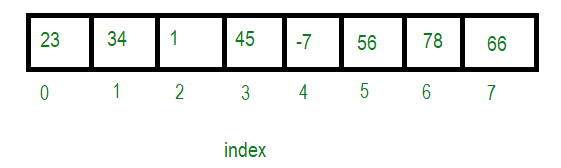Below are some advantages of the array:

• In an array, accessing an element is very easy by using the index number.
• The search process can be applied to an array easily.
• 2D Array is used to represent matrices.
• For any reason a user wishes to store multiple values of similar type then the Array can be used and utilized efficiently.

Now let’s see some disadvantages of the array and how to overcome it:

Array size is fixed: The array is static, which means its size is always fixed. The memory which is allocated to it cannot be increased or decreased. Below is the program for the same:

## C

 `// C program to illustrate that the ` `// array size is fixed ` `#include ` ` `  `// Driver Code ` `int` `main() ` `{ ` `    ``int` `arr; ` ` `  `    ``// Assign values to array ` `    ``arr = 5; ` `    ``arr = 6; ` `    ``arr = -9; ` ` `  `    ``// Print array element at index 0 ` `    ``printf``(``"Element at index 0"` `           ``" is %d\n"``, ` `           ``arr); ` ` `  `    ``// Print array element at index 11 ` `    ``printf``(``"Element at index 11"` `           ``" is %d"``, ` `           ``arr); ` ` `  `    ``return` `0; ` `} `

Output:

```Element at index 0 is 5
Element at index 11 is -1176897384
```

Explanation: In the above program the array of size 10 is declared and the value is assigned at a particular index. But when the value at index 11 is printed then it prints the garbage value because the array was accessed out of the bound index. In some compiler, it gives error as “Array Index Out Of Bound.”.

How to overcome: To overcome that problem use Dynamic Memory Allocation like malloc(), calloc(). It also helps us to deallocates the memory using the free() method which helps to reduce wastage of memory by releasing it. Below is the program for the same:

## C

 `// C program to illustrate the use of ` `// array using Dynamic Memory Allocation ` `#include ` `#include ` ` `  `// Driver Code ` `int` `main() ` `{ ` `    ``// Pointer will hold the base address ` `    ``int``* ptr; ` `    ``int` `n = 10; ` ` `  `    ``// Dynamically allocates memory ` `    ``// using malloc() function ` `    ``ptr = (``int``*)``malloc``(n * ``sizeof``(``int``)); ` ` `  `    ``// Assign values to the array ` `    ``for` `(``int` `i = 0; i < n; i++) { ` `        ``ptr[i] = i + 1; ` `    ``} ` ` `  `    ``// Print the array ` `    ``printf``(``"The elements are: "``); ` ` `  `    ``for` `(``int` `i = 0; i < n; i++) { ` `        ``printf``(``"%d "``, ptr[i]); ` `    ``} ` ` `  `    ``// Free the dynamically ` `    ``// allocated memory ` `    ``free``(ptr); ` ` `  `    ``return` `0; ` `} `

Output:

```The elements are: 1 2 3 4 5 6 7 8 9 10
```

Array is homogeneous:The array is homogeneous, i.e., only one type of value can be store in the array. For example, if an array type “int“, can only store integer elements and cannot allow the elements of other types such as double, float, char so on. Below is the program for the same:

## C

 `// C++ program to illustrate that ` `// the array is homogeneous ` `#include ` ` `  `// Driver Code ` `int` `main() ` `{ ` `    ``// Below declaration will give ` `    ``// Compilation Error ` `    ``int` `a = { 0, 1, 2, ``"string"``, 9, 4.85 }; ` ` `  `    ``return` `0; ` `} `

Output:

```100
547.000000
Ram
```

Output: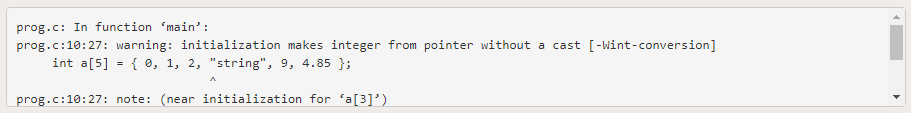Explanation: The above code gives “Compilation Error” as an integer type array is assigned value to a string and float type.

How to overcome: To overcome that problem, the idea is to structure, where it can store non-homogeneous (heterogeneous) value. Below is the program for the same:

## C

 `// C program to illustrate the use of ` `// structure to store heterogeneous ` `// variables ` `#include ` ` `  `// Structure students ` `struct` `student { ` ` `  `    ``int` `student_id; ` `    ``float` `marks; ` `    ``char` `name; ` `}; ` ` `  `// Driver Code ` `int` `main() ` `{ ` `    ``// Structure variable s1 ` `    ``struct` `student s1 = { 100, 547, ``"Ram"` `}; ` ` `  `    ``// Accessing structure members ` `    ``// using structure pointer ` `    ``printf``(``"%d\n"``, s1.student_id); ` `    ``printf``(``"%f\n"``, s1.marks); ` ` `  `    ``for` `(``int` `i = 0; ` `         ``s1.name[i] != ``'\0'``; i++) { ` `        ``printf``(``"%c"``, s1.name[i]); ` `    ``} ` ` `  `    ``return` `0; ` `} `

Output:

```100
547.000000
Ram
```

Array is Contiguous blocks of memory: The array stores data in contiguous(one by one) memory location. Below is the representation of the same: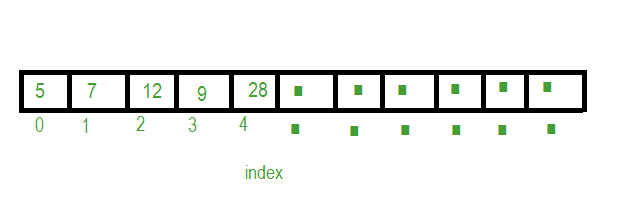How to overcome: To overcome the sequential access to the array, the idea is to use the Linked list. In a Linked list, the elements are not stored in contiguous memory locations. Below is the representation of the same: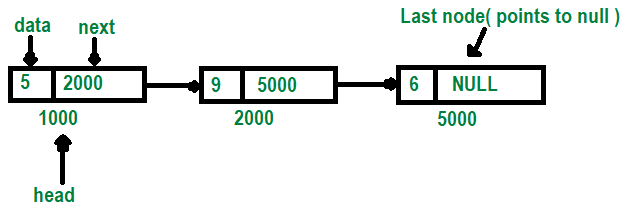Insertion and deletion are not easy in Array: The operation insertion and deletion over an array are problematic as to insert or delete anywhere in the array, it is necessary to traverse the array and then shift the remaining elements as per the operation. This operation cost is more.

Example: For inserting 22 in 3rd position of the array then below are the steps: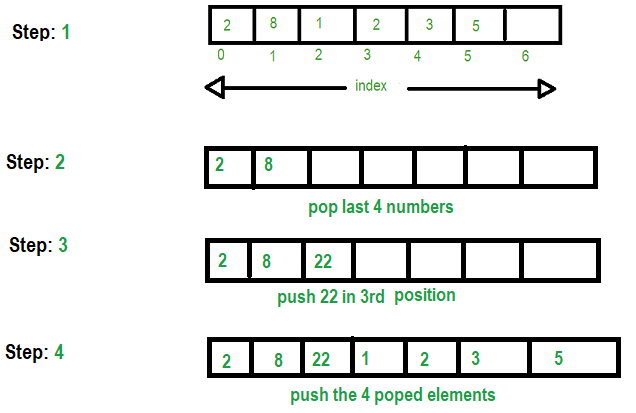Below is the program to illustrate the same:

## C

 `// C Program to insert an element at ` `// a specific position in an array ` `#include ` ` `  `// Driver Code ` `int` `main() ` `{ ` `    ``int` `arr = { 0 }; ` `    ``int` `i, x, pos, n = 10; ` ` `  `    ``// Initial array of size 10 ` `    ``for` `(i = 0; i < 10; i++) { ` `        ``arr[i] = i + 1; ` `    ``} ` ` `  `    ``// Print the original array ` `    ``for` `(i = 0; i < n; i++) { ` `        ``printf``(``"%d "``, arr[i]); ` `    ``} ` `    ``printf``(``"\n"``); ` ` `  `    ``// Element to be inserted ` `    ``x = 50; ` ` `  `    ``// Position at which element ` `    ``// is to be inserted ` `    ``pos = 5; ` ` `  `    ``printf``(``"Array after inserting %d"` `           ``" at position %d\n"``, ` `           ``x, pos); ` ` `  `    ``// Increase the size by 1 ` `    ``n++; ` ` `  `    ``// Shift elements forward ` `    ``for` `(i = n - 1; i >= pos; i--) { ` ` `  `        ``arr[i] = arr[i - 1]; ` `    ``} ` ` `  `    ``// Insert x at pos ` `    ``arr[pos - 1] = x; ` ` `  `    ``// Print the updated array ` `    ``for` `(i = 0; i < n; i++) { ` `        ``printf``(``"%d "``, arr[i]); ` `    ``} ` ` `  `    ``return` `0; ` `} `

Output:

```1 2 3 4 5 6 7 8 9 10
Array after inserting 50 at position 5
1 2 3 4 50 5 6 7 8 9 10
```

How to overcome: To overcome the above problem using a Linked List. Below is the representation of the same: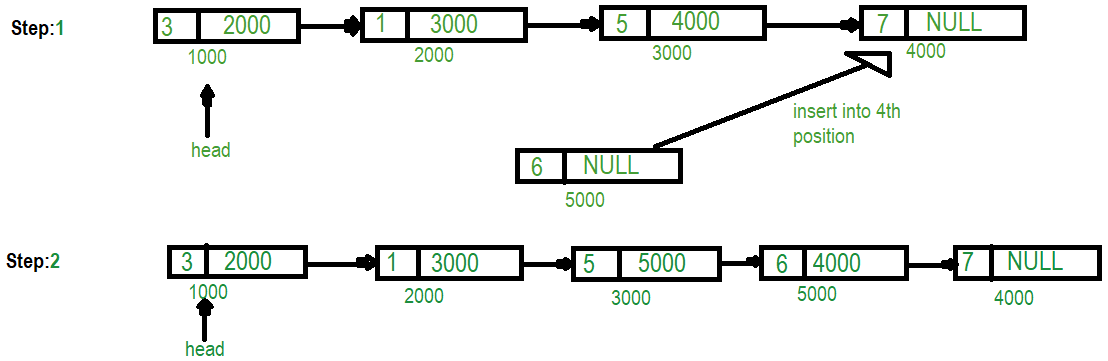Below is the program to implement the same:

## C

 `// C program to insert an element at ` `// a position using linked list ` `#include ` `#include ` ` `  `// Structure for the linked list ` `struct` `node { ` `    ``int` `data; ` `    ``struct` `node* next; ` `}; ` ` `  `// head Node ` `struct` `node* head; ` ` `  `// Function to insert any element ` `// at the end of the linked list ` `int` `insert_last(``int` `k) ` `{ ` `    ``struct` `node *ptr, *s; ` `    ``ptr = (``struct` `node*) ` `        ``malloc``(``sizeof``(``struct` `node)); ` `    ``ptr->data = k; ` `    ``ptr->next = NULL; ` ` `  `    ``// If head is NULL ` `    ``if` `(head == NULL) { ` `        ``head = ptr; ` `    ``} ` ` `  `    ``// Else ` `    ``else` `{ ` ` `  `        ``s = head; ` ` `  `        ``// Traverse the LL to last ` `        ``while` `(s->next != NULL) { ` `            ``s = s->next; ` `        ``} ` `        ``s->next = ptr; ` `    ``} ` `} ` ` `  `// Function to display linked list ` `void` `display() ` `{ ` `    ``struct` `node* s; ` ` `  `    ``// Store the head ` `    ``s = head; ` ` `  `    ``// Traverse till s is NULL ` `    ``while` `(s != NULL) { ` ` `  `        ``// Print the data ` `        ``printf``(``"%d "``, s->data); ` `        ``s = s->next; ` `    ``} ` `    ``printf``(``"\n"``); ` `} ` ` `  `// Function to insert any element at ` `// the given position of linked list ` `int` `insert_position(``int` `a, ``int` `b) ` `{ ` `    ``int` `f = 0, i; ` `    ``struct` `node *ptr, *s; ` ` `  `    ``// Allocate new Node ` `    ``ptr = (``struct` `node*) ` `        ``malloc``(``sizeof``(``struct` `node)); ` `    ``ptr->data = a; ` ` `  `    ``// If first position ` `    ``if` `(b == 1) { ` `        ``ptr->next = head; ` `        ``head = ptr; ` `    ``} ` ` `  `    ``// Otherwise ` `    ``else` `{ ` `        ``s = head; ` ` `  `        ``// Move to (b - 1) position ` `        ``for` `(i = 0; i < b - 2; i++) { ` `            ``s = s->next; ` `        ``} ` ` `  `        ``// Assign node ` `        ``ptr->next = s->next; ` `        ``s->next = ptr; ` `    ``} ` ` `  `    ``return` `0; ` `} ` ` `  `// Driver Code ` `int` `main() ` `{ ` `    ``// Given Linked List ` `    ``insert_last(3); ` `    ``insert_last(1); ` `    ``insert_last(5); ` `    ``insert_last(7); ` ` `  `    ``printf``(``"Current Linked List is: "``); ` ` `  `    ``// Display the LL ` `    ``display(); ` ` `  `    ``// Insert 6 at position 4 ` `    ``insert_position(6, 4); ` `    ``printf``(``"\n Linked List after insert"` `           ``" 6 in 4th position: "``); ` ` `  `    ``// Display the LL ` `    ``display(); ` ` `  `    ``return` `0; ` `} `

Output:

```Current Linked List is: 3 1 5 7

Linked List after insert 6 in 4th position: 3 1 5 6 7
```

Attention reader! Don’t stop learning now. Get hold of all the important DSA concepts with the DSA Self Paced Course at a student-friendly price and become industry ready.

My Personal Notes arrow_drop_upCheck out this Author's contributed articles.

If you like GeeksforGeeks and would like to contribute, you can also write an article using contribute.geeksforgeeks.org or mail your article to contribute@geeksforgeeks.org. See your article appearing on the GeeksforGeeks main page and help other Geeks.

Please Improve this article if you find anything incorrect by clicking on the "Improve Article" button below.